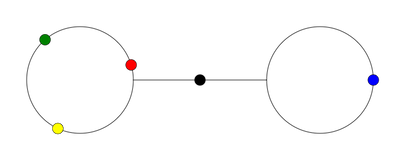## Configuration Spaces of Graphs### Configuration spaces

Definition: Let $X$ be a topological space and $n\in\mathbb{N}$, then the configuration space of $n$ ordered particles in $X$ is defined as the set $$\mathrm{Conf}_n(X) = \{ (x_1, \ldots, x_n) \,|\, \text{x_i \neq x_j for i\neq j} \}$$ endowed with the subspace topology of $X^n$.
The symmetric group $\Sigma_n$ acts by permuting indices.

### Graphs

Definition: A graph $G$ is a 1-dimensional CW-complex.
It consists of sets
• $V(G)$ of 0-dimensional cells called vertices and
• $E(G)$ of 1-dimensional cells called edges.

### Main result

Theorem (Chettih-L)
If $T$ is a finite tree, then $H_\bullet (\mathrm{Conf}_n(T); \mathbb{Z})$ is free and generated by products of disjoint basic classes.
Definition: A basic class is a class in the image of the map $$H_1(\mathrm{Conf}_n(\iota))\colon H_1(\mathrm{Conf}_n(G)) \to H_1(\mathrm{Conf}_n(T))$$ induced by a piecewise linear embedding $\iota\colon G\hookrightarrow T$, where $G$ is a star graph or the H-shaped graph.

### Main result

Theorem (Chettih-L)
If $T$ is a finite tree, then $H_\bullet (\mathrm{Conf}_n(T); \mathbb{Z})$ is free and generated by products of disjoint basic classes.

### A combinatorial model

We will work with a combinatorial cube complex model of $\mathrm{Conf}_n(G)$, analogous to Świątkowski's model in the unordered case.
Its zero dimensional cubes are given by configurations where the particles on each edge are equidistant.

### A combinatorial model

One dimensional cubes move one particle from a vertex to an edge.
Move mouse along the interval

### A combinatorial model

A two dimensional cube moves two particles from two distinct vertices onto edges.
Move mouse along the square

### A combinatorial model

Theorem (L)
If $G$ is a finite graph, then $\mathrm{Conf}_n(G)$ deformation retracts to a cube complex of dimension $\mathrm{min}\{n, |V|\}$, where $V$ is the set of essential vertices.
Therefore, for graphs with exactly one essential vertex the combinatorial model is itself a graph and it is easy to determine its homology.
Example: $\mathrm{Conf}_2(Y)\simeq S^1$
Move mouse along the combinatorial model

### A combinatorial model

If the number of particles is at least $2|V|$, then the dimension of this combinatorial model is equal to the homological dimension of the space.

### Configuration spaces with sinks

The map collapsing a subgraph $$G \to G/H$$ does not induce a map on configuration spaces.

### Configuration spaces with sinks

To solve this, we introduce sinks to our graphs. Sinks are vertices where particles are allowed to collide.
Definition: For a graph $G$ and a subset $Z$ of the vertices, define $$\mathrm{Conf}_n(G,Z) := \{ (x_1,\ldots,x_n) | \text{x_i\neq x_j or x_i\in Z} \}.$$

### Configuration spaces with sinks

Collapsing a subgraph $H$ of $G$ now induces a map $$\mathrm{Conf}_n(G) \to \mathrm{Conf}_n(G/H, H/H).$$
Collapsing and computing the homology of small graphs with sinks allows us to compute the homology of configurations in trees.

### Configuration spaces with sinks

Theorem (Chettih-L)
If $G$ is a finite graph and $Z$ a subset of the vertices, then $\mathrm{Conf}_n(G,Z)$ deformation retracts to a cube complex of dimension $\mathrm{min}\{n, |V| + |E_\mathrm{sink}| \}$, where $V$ is the set of essential vertices and $E_\mathrm{sink}$ is the set of edges incident to two sinks.
Example: $\mathrm{Conf}_2([0,1], \{0,1\})\simeq S^1$
Move mouse along the combinatorial model

### Explicit calculations

For star graphs, the combinatorial model is good enough to compute the homology:
Theorem (Ghrist)
For $n\ge 1$ and $k\ge 3$ we have $$\tilde{H}_q(\mathrm{Conf}_n(\mathrm{Star}_k)) \cong \begin{cases} \mathbb{Z}^{r(k,n)} & \text{q = 1,}\\ 0 & \text{else,} \end{cases}$$ where $r(k,n) = 1 + \frac{(n+k-2)!}{(k-1)!} (n(k-2) - k + 1)$.

### Explicit calculations

For the interval with sinks, we get:
Proposition (Chettih-L)
For $n\ge 1$ we have $$\tilde{H}_q\left(\mathrm{Conf}_n([0,1], \{0\})\right) =0,$$ and $$\tilde{H}_q\left(\mathrm{Conf}_n([0,1], \{0,1\})\right) \cong \begin{cases} \mathbb{Z}^{r(n)} & \text{q = 1,}\\ 0 & \text{else,} \end{cases}$$ where $r(n) = 1 + (n-2) 2^{n-1}$.

### Explicit calculations

It turns out that these are the only building blocks we need to describe the homology $H_*(\mathrm{Conf}_n(T))$ for any finite tree $T$.
To illustrate the approach of our proof, we will now compute $H_*(\mathrm{Conf}_n(\mathrm{H}))$.

### The Mayer-Vietoris spectral sequence

Let $n\ge 1$, $G$ a finite graph, $Z$ a subset of the vertices and $\{V_i\}_{i\in I}$ an open cover of $G$.
For each map $$\phi\colon \{1,\ldots,n\}\to I$$ define the open subset $U_\phi$ of $\mathrm{Conf}_n(G,Z)$ as $$U_\phi = \bigcap_{1\le j\le n} \pi_j^{-1}( V_{\phi(j)}),$$ where $\pi_j\colon\mathrm{Conf}_n(G,Z)\to G$ projects to the $j$-th particle.
This defines an open cover $\{ U_\phi \}$ of $\mathrm{Conf}_n(G,Z)$.

### The Mayer-Vietoris spectral sequence

Associated to this open cover $\{ U_\phi \}$ of $\mathrm{Conf}_n(G,Z)$ there is the Mayer-Vietoris spectral sequence given by $$E^1_{p,q} = \bigoplus_{\phi_0,\ldots,\phi_p} H_q( U_{\phi_0} \cap\cdots\cap U_{\phi_p})$$ converging to the homology $H_*(\mathrm{Conf}_n(G,Z))$.
Instead of computing the pages directly, we compare the spectral sequences for different graphs and different sets of sinks.

### The H-shaped graph

As open cover for the graph $\mathrm{H}$ we take $V_1$ and $V_2$ defined as follows:
This defines a spectral sequence $E^*_{\bullet,\bullet}$.

### The H-shaped graph

Each intersection of sets $U_\phi$ is a disjoint union of spaces of the form $$\mathrm{Conf}_{S_1}(V_1) \times \mathrm{Conf}_{S_I}(V_1\cap V_2) \times \mathrm{Conf}_{S_2}(V_2)$$ for some $S_1\sqcup S_I \sqcup S_2 = \{1,\ldots,n\}$.

### The H-shaped graph

The connected components of $\mathrm{Conf}_{S_I}(V_1\cap V_2)$ are contractible and determined by the order of the particles $S_I$. By the Künneth theorem, the $q$-th homology of those products is given by $$\bigoplus_{q_1+q_2=q} H_{q_1}( \mathrm{Conf}_{S_1}(V_1) )\otimes H_{q_2}( \mathrm{Conf}_{S_2}(V_2)).$$

### The H-shaped graph

This implies that only the three bottom rows of $E^1_{\bullet,\bullet}$ are non-trivial.

### The H-shaped graph

We now compute the bottom row $E^2_{\bullet,0}$.
Entries are sums of modules $$H_0(\mathrm{Conf}_{S^j_1}(V_1))\otimes H_0(\mathrm{Conf}_{S^j_2}(V_2))$$ These groups don't change if we turn the vertices into sinks.
$\cong H_\bullet(\mathrm{Conf}(\mathrm{H},V(\mathrm{H}))$
$\cong H_\bullet(\mathrm{Conf}([0,1],\{0,1\}))$

### The H-shaped graph

We now compute the first row $E^2_{\bullet,1}$.
Entries are sums of modules $$H_1(\mathrm{Conf}_{S^j_1}(V_1))\otimes H_0(\mathrm{Conf}_{S^j_2}(V_2))$$ and $$H_0(\mathrm{Conf}_{S^j_1}(V_1))\otimes H_1(\mathrm{Conf}_{S^j_2}(V_2))$$ These groups don't change if we turn one of the vertices into a sink.

### The H-shaped graph

This describes the infinity page.

### The H-shaped graph

This gives a generating set of $H_*(\mathrm{Conf}_n(\mathrm{H}))$ in terms of classes in the configuration spaces of the $\mathrm{Y}$-graph and the interval with two sinks.
The general proof proceeds in the same way by glueing star graphs to trees.